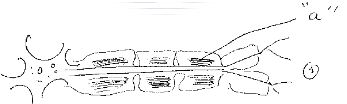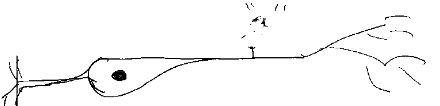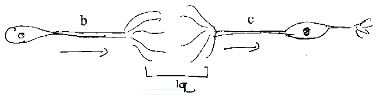1.

Which of the following option is not correct for the region labelled as "a" in the given diagram.A. White and fatty compound

B. It is Conductive

C. Region without it called node of Ranvier

D. It is responsible for saltatary conduction

Answer : Option B

2.  Which of the given option is correct for autonomous nervous system ?

A. In it medullary sheath is very well developed

B. Node of Ranvier is present in it.

C. It is part of CNS

D. It's nerve do not travel for longer distance in body

Answer : Option D

3.

What does given diagram show ? where is it found ?A. Unipolarneuron in spinal cord

B. Bipolar neuron ; in eye of human

C. Unipolar neuron; In embroynic stage

D. Unipolar neuron in eye of human

Answer : Option B

4.

What does a and b indicate in the given diagram ?A. Synaptic cleft

B. Synase between axon

C. Synapse between axon and dendron

D. Synapse - between two dendrite

Answer : Option C

5.  What does "b" and "c" indicate in the given diagram in question no 4?

A. b = Synapse; c = dendrites

B. c = Synapse; c = Axon fibres

C. b = axon c = dendrit

D. b = Synaptic bulb; c = Motor nerve

Answer : Option C

6.

What dose "a" and "b" indicate in the given diagram ?A. a = Dendrite; b = Nissl's granules

B. a = Axon fibres ; b = Nucleus

C. a = Axon fibres ; b = Mitochondria

D. None of the given

Answer : Option D

7.  What is correct in context with neuron ?

A. Nissl's granule present in it are acidic

B. It's cytoplam is restricted to only cyton

C. Each neuron has one axon and one dendron

D. Cyton possesses large nucleus

Answer : Option D

8.  In which animal nervous system is in the form of nerve-net ?

A. Leucosolania

B. Liver-Fluke

C. Planaria

D. Hydra

Answer : Option D

9.  In which animal neverous system consist of brain, ganglion and nerve fiber ?

A. Tape-worm

B. Liver-Fluke

C. Ascaris

D. (d) Allof the given

Answer : Option C

10.

which option shows correctly matched pairs for the column I and Column II ?

Colum-I                                    Column-II

(P)Unipolar neron                      (i)Retina

(Q)Bipolar neuron                     (ii)Cerebral hemisphere

(R)Multipolar neuron                (iii)Embroyonic stage

A. (P-iii), (Q-i), (R-ii)

B. (P-ii), (Q-i), (R-iii)

C. (P-iii), (Q-ii), (R-i)

D. (P-ii), (Q-iii), (R-ii)

Answer : Option A